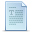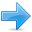# Templates

### Function templates

Function templates are special functions that can operate with generic types. This allows us to create a function template whose functionality can be adapted to more than one type or class without repeating the entire code for each type.

In C++ this can be achieved using template parameters. A template parameter is a special kind of parameter that can be used to pass a type as argument: just like regular function parameters can be used to pass values to a function, template parameters allow to pass also types to a function. These function templates can use these parameters as if they were any other regular type.

The format for declaring function templates with type parameters is:

template <class identifier> function_declaration;
template <typename identifier> function_declaration;

The only difference between both prototypes is the use of either the keyword class or the keyword typename. Its use is indistinct, since both expressions have exactly the same meaning and behave exactly the same way.

For example, to create a template function that returns the greater one of two objects we could use:

 ``1234`` ``````template myType GetMax (myType a, myType b) { return (a>b?a:b); }``````

Here we have created a template function with myType as its template parameter. This template parameter represents a type that has not yet been specified, but that can be used in the template function as if it were a regular type. As you can see, the function template GetMax returns the greater of two parameters of this still-undefined type.

To use this function template we use the following format for the function call:

function_name <type> (parameters);

For example, to call GetMax to compare two integer values of type int we can write:

 ``12`` ``````int x,y; GetMax (x,y);``````

When the compiler encounters this call to a template function, it uses the template to automatically generate a function replacing each appearance of myType by the type passed as the actual template parameter (int in this case) and then calls it. This process is automatically performed by the compiler and is invisible to the programmer.

Here is the entire example:

 ``````// function template #include using namespace std; template T GetMax (T a, T b) { T result; result = (a>b)? a : b; return (result); } int main () { int i=5, j=6, k; long l=10, m=5, n; k=GetMax(i,j); n=GetMax(l,m); cout << k << endl; cout << n << endl; return 0; }`````` ```6 10```

In this case, we have used T as the template parameter name instead of myType because it is shorter and in fact is a very common template parameter name. But you can use any identifier you like.

In the example above we used the function template GetMax() twice. The first time with arguments of type int and the second one with arguments of type long. The compiler has instantiated and then called each time the appropriate version of the function.

As you can see, the type T is used within the GetMax() template function even to declare new objects of that type:

 ``1`` ``T result;``

Therefore, result will be an object of the same type as the parameters a and b when the function template is instantiated with a specific type.

In this specific case where the generic type T is used as a parameter for GetMax the compiler can find out automatically which data type has to instantiate without having to explicitly specify it within angle brackets (like we have done before specifying <int> and <long>). So we could have written instead:

 ``12`` ``````int i,j; GetMax (i,j);``````

Since both i and j are of type int, and the compiler can automatically find out that the template parameter can only be int. This implicit method produces exactly the same result:

 ``````// function template II #include using namespace std; template T GetMax (T a, T b) { return (a>b?a:b); } int main () { int i=5, j=6, k; long l=10, m=5, n; k=GetMax(i,j); n=GetMax(l,m); cout << k << endl; cout << n << endl; return 0; }`````` ```6 10```

Notice how in this case, we called our function template GetMax() without explicitly specifying the type between angle-brackets <>. The compiler automatically determines what type is needed on each call.

Because our template function includes only one template parameter (class T) and the function template itself accepts two parameters, both of this T type, we cannot call our function template with two objects of different types as arguments:

 ``123`` ``````int i; long l; k = GetMax (i,l);``````

This would not be correct, since our GetMax function template expects two arguments of the same type, and in this call to it we use objects of two different types.

We can also define function templates that accept more than one type parameter, simply by specifying more template parameters between the angle brackets. For example:

 ``1234`` ``````template T GetMin (T a, U b) { return (a

In this case, our function template GetMin() accepts two parameters of different types and returns an object of the same type as the first parameter (T) that is passed. For example, after that declaration we could call GetMin() with:

 ``123`` ``````int i,j; long l; i = GetMin (j,l);``````

or simply:

 ``1`` ``i = GetMin (j,l);``

even though j and l have different types, since the compiler can determine the appropriate instantiation anyway.

### Class templates

We also have the possibility to write class templates, so that a class can have members that use template parameters as types. For example:

 ``123456789`` ``````template class mypair { T values ; public: mypair (T first, T second) { values=first; values=second; } };``````

The class that we have just defined serves to store two elements of any valid type. For example, if we wanted to declare an object of this class to store two integer values of type int with the values 115 and 36 we would write:

 ``1`` ``mypair myobject (115, 36);``

this same class would also be used to create an object to store any other type:

 ``1`` ``mypair myfloats (3.0, 2.18);``

The only member function in the previous class template has been defined inline within the class declaration itself. In case that we define a function member outside the declaration of the class template, we must always precede that definition with the template <...> prefix:

 ``123456789101112131415161718192021222324252627282930313233343536373839404142434445464748495051525354555657585960616263646566676869707172737475767778798081828384858687888990919293949596979899100`` ``````// class templates #include using namespace std; template class mypair { T a, b; public: mypair (T first, T second) {a=first; b=second;} T getmax (); }; template T mypair::getmax () { T retval; retval = a>b? a : b; return retval; } int main () { mypair myobject (100, 75); cout << myobject.getmax(); return 0; }`````` `100`

Notice the syntax of the definition of member function getmax:

 ``12`` ``````template T mypair::getmax ()``````

Confused by so many T's? There are three T's in this declaration: The first one is the template parameter. The second T refers to the type returned by the function. And the third T (the one between angle brackets) is also a requirement: It specifies that this function's template parameter is also the class template parameter.

### Template specialization

If we want to define a different implementation for a template when a specific type is passed as template parameter, we can declare a specialization of that template.

For example, let's suppose that we have a very simple class called mycontainer that can store one element of any type and that it has just one member function called increase, which increases its value. But we find that when it stores an element of type char it would be more convenient to have a completely different implementation with a function member uppercase, so we decide to declare a class template specialization for that type:

 ``````// template specialization #include using namespace std; // class template: template class mycontainer { T element; public: mycontainer (T arg) {element=arg;} T increase () {return ++element;} }; // class template specialization: template <> class mycontainer { char element; public: mycontainer (char arg) {element=arg;} char uppercase () { if ((element>='a')&&(element<='z')) element+='A'-'a'; return element; } }; int main () { mycontainer myint (7); mycontainer mychar ('j'); cout << myint.increase() << endl; cout << mychar.uppercase() << endl; return 0; }`````` ```8 J```

This is the syntax used in the class template specialization:

 ``1`` ``template <> class mycontainer { ... };``

First of all, notice that we precede the class template name with an empty template<> parameter list. This is to explicitly declare it as a template specialization.

But more important than this prefix, is the <char> specialization parameter after the class template name. This specialization parameter itself identifies the type for which we are going to declare a template class specialization (char). Notice the differences between the generic class template and the specialization:

 ``12`` ``````template class mycontainer { ... }; template <> class mycontainer { ... };``````

The first line is the generic template, and the second one is the specialization.

When we declare specializations for a template class, we must also define all its members, even those exactly equal to the generic template class, because there is no "inheritance" of members from the generic template to the specialization.

### Non-type parameters for templates

Besides the template arguments that are preceded by the class or typename keywords , which represent types, templates can also have regular typed parameters, similar to those found in functions. As an example, have a look at this class template that is used to contain sequences of elements:

 ``````// sequence template #include using namespace std; template class mysequence { T memblock [N]; public: void setmember (int x, T value); T getmember (int x); }; template void mysequence::setmember (int x, T value) { memblock[x]=value; } template T mysequence::getmember (int x) { return memblock[x]; } int main () { mysequence myints; mysequence myfloats; myints.setmember (0,100); myfloats.setmember (3,3.1416); cout << myints.getmember(0) << '\n'; cout << myfloats.getmember(3) << '\n'; return 0; }`````` ```100 3.1416 ```

It is also possible to set default values or types for class template parameters. For example, if the previous class template definition had been:

 ``1`` ``template class mysequence {..};``

We could create objects using the default template parameters by declaring:

 ``1`` ``mysequence<> myseq;``

Which would be equivalent to:

 ``1`` ``mysequence myseq;``

### Templates and multiple-file projects

From the point of view of the compiler, templates are not normal functions or classes. They are compiled on demand, meaning that the code of a template function is not compiled until an instantiation with specific template arguments is required. At that moment, when an instantiation is required, the compiler generates a function specifically for those arguments from the template.

When projects grow it is usual to split the code of a program in different source code files. In these cases, the interface and implementation are generally separated. Taking a library of functions as example, the interface generally consists of declarations of the prototypes of all the functions that can be called. These are generally declared in a "header file" with a .h extension, and the implementation (the definition of these functions) is in an independent file with c++ code.

Because templates are compiled when required, this forces a restriction for multi-file projects: the implementation (definition) of a template class or function must be in the same file as its declaration. That means that we cannot separate the interface in a separate header file, and that we must include both interface and implementation in any file that uses the templates.

Since no code is generated until a template is instantiated when required, compilers are prepared to allow the inclusion more than once of the same template file with both declarations and definitions in a project without generating linkage errors.Previous:PolymorphismIndexNext:Namespaces